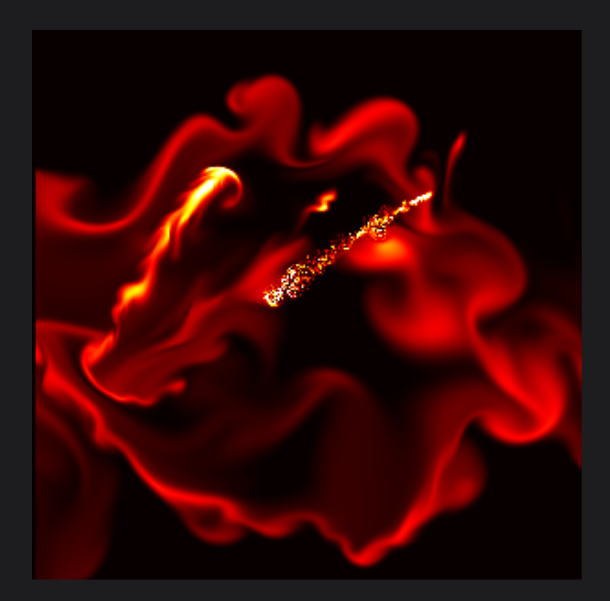HOME  >   HTML5 CANVAS  >   How HTML5 Canvas Implements Foggy Flame Animation
How HTML5 Canvas Implements Foggy Flame Animation
BY Craig7 Dec,2020
Tags:

We can draw a lot of graphics on Canvas, and we can also use HTML technology to make some complex animations on Canvas. We have also shared some flame animations based on HTML5 Canvas before. For example, this HTML5 Canvas magical flame text effect and shared earlier HTML5 Canvas flame burning animation. This time we bring you another flame animation based on HTML5 Canvas. You can click and drag on the Canvas drawing board with the mouse to form a fog-like flame effect, and the flame will gradually spread like haze.HTML Body Code
```function set_bnd (b, x){
for(i=1; i<=height; i++) {
x[IX(0, i)]      = 0;
x[IX(width+1,i)] = 0;

x[IX(i, 0)]      = 0;
x[IX(i,width+1)] = 0;
}

x[IX(0, 0)] = 0.5*(x[IX(1, 0)]+x[IX(0, 1)]);
x[IX(0,width+1)] = 0.5*(x[IX(1,width+1)]+x[IX(0, height)]);
x[IX(width+1, 0)] = 0.5*(x[IX(width, 0)]+x[IX(width+1,1)]);
x[IX(width+1,height+1)] = 0.5*(x[IX(width,height+1)]+x[IX(width+1,width)]);
}

function diffuse(b, x, x0, diff, dt){

var a=dt*diff*width*width;

for(k=0; k<iterations; k++) {
for(i=1; i<=width; i++) {
for(j=1; j<=height; j++) {
x[IX(i,j)] =(x0[IX(i,j)] + a*(x[IX(i-1,j)]+x[IX(i+1,j)]+x[IX(i,j-1)]+x[IX(i,j+1)]))/(1+4*a);
}
}
set_bnd(b, x);
}
}                        ```
Css Code
```body {
background-color: rgb(29,29,32);
margin: 0;
overflow: hidden;
background-repeat: no-repeat;
}
.label {
position: absolute;
top: 0;
left: 0;
color: #eee;
font-size: 13px;
background-color: rgba(0, 0, 0, .15);
}
.instructions {
position: absolute;
bottom: 0%;
left: 0;